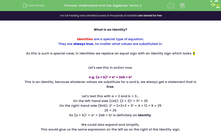# Understand and Use Algebraic Terms 2

In this worksheet, students will learn to recognise identities and use their unique properties to fine the value of terms in expressions.Key stage:  KS 4

Year:  GCSE

GCSE Subjects:   Maths

GCSE Boards:   Pearson Edexcel, OCR, Eduqas, AQA,

Curriculum topic:   Algebra

Curriculum subtopic:   Notation, Vocabulary and Manipulation Algebraic Formulae

Difficulty level:#### Worksheet Overview

​What is an identity?​

Identities are a special type of equation.

They are always true, no matter what values are substituted in.

As this is such a special case, in identities we replace an equal sign with an identity sign which looks:

Let's see this in action now.

e.g. (a + b)² = a² + 2ab + b²

This is an identity, because whatever values we substitute for a and b, we always get a statement that is true.

Let's test this with a = 2 and b = 3...

On the left-hand side (LHS): (2 + 3)² = 5² = 25

On the right-hand side (RHS): 2² + 2×2×3 + 3² = 4 + 12 + 9 = 25

25 = 25

So (a + b)² = a² + 2ab + b² is definitely an identity.

We could also expand and simplify.

This would give us the same expression on the left as on the right of the identity sign.

How to use an identity?​

At GCSE level, you might be asked to use the properties of identities to find missing coefficients.

e.g. 5ax + 8 + 3(x - d) 18x + 14

Find the values of a and d.

The highlighted sign is an identity sign, so this means that the LHS and the RHS will simplify to exactly the same expressions.

Let's expand and simplify the LHS first:

5ax + 8 + 3(x - d) = 5ax + 8 + 3x - 3d

Now let's remove x from the RHS, by factorising the left-hand terms with x:

5ax + 3x + 8 - 3d = x(5a + 3) + 8 - 3d

Therefore, 5a + 3 = 18 and 8 - 3d = 14.

So a = 3, d = -2

In this activity, we will learn to recognise identities and use their unique properties to fine the value of terms in expressions.

### What is EdPlace?

We're your National Curriculum aligned online education content provider helping each child succeed in English, maths and science from year 1 to GCSE. With an EdPlace account you’ll be able to track and measure progress, helping each child achieve their best. We build confidence and attainment by personalising each child’s learning at a level that suits them.

Get started